# HiSET Mathematics: Polynomials Chapter Exam

Exam Instructions:

Choose your answers to the questions and click 'Next' to see the next set of questions. You can skip questions if you would like and come back to them later with the yellow "Go To First Skipped Question" button. When you have completed the practice exam, a green submit button will appear. Click it to see your results. Good luck!

### Page 1

#### Question 1 1. The following graph is of a polynomial function of degree 2. Are the solutions of this function real or imaginary and why?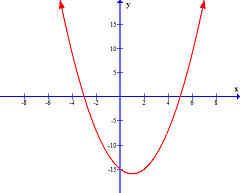#### Question 2 2. The possible rational zeros for the following function are +/-1 and +/-2. Which synthetic division problem shows that 2 is NOT a rational zero of the function?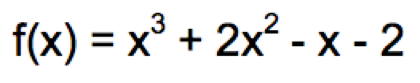### Page 2

#### Question 7 7. Which of the following answer choices is a possible rational zero for the following function?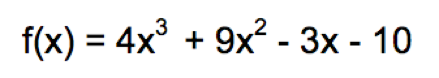#### Question 10 10. Divide using long division.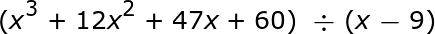### Page 3

#### Question 12 12. Divide using long division.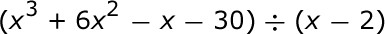#### Question 13 13. The graph of the function f(x) = x^3 - 4x^2 - 2x + 8 is shown below. This function has only one rational zero. Use the rational zeros theorem and the graph to determine the value of the rational zero.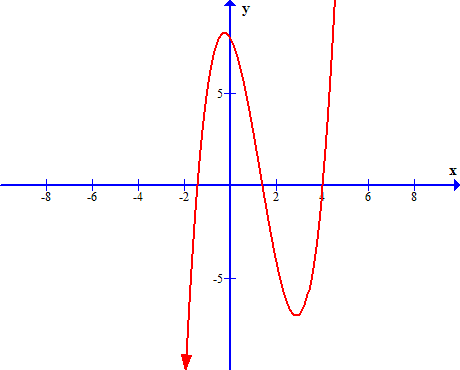#### Question 14 14. The following graph is of a polynomial function of degree 4. Which of the following best describes the solutions?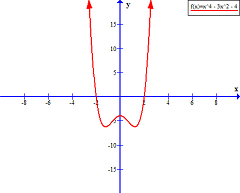### Page 4

#### Question 18 18. Divide using long division.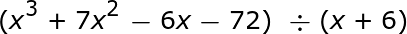### Page 5

#### Question 21 21. Which answer choice is not a possible rational zero for the following function?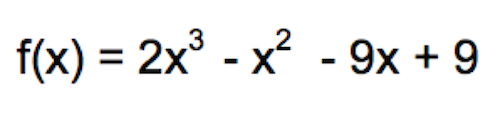### Page 6

#### Question 29 29. Divide using long division.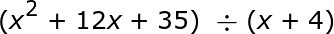#### Question 30 30. Divide using long division.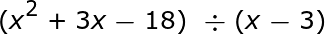#### HiSET Mathematics: Polynomials Chapter Exam Instructions

Choose your answers to the questions and click 'Next' to see the next set of questions. You can skip questions if you would like and come back to them later with the yellow "Go To First Skipped Question" button. When you have completed the practice exam, a green submit button will appear. Click it to see your results. Good luck!

Support